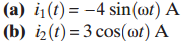Chat Now

# Transform the following sinusoids into phasor form and draw a phasor diagram. Use the additive...

### Transform the following sinusoids into phasor form and draw a phasor diagram. Use the additive...

Transform the following sinusoids into phasor form and draw a phasor diagram. Use the additive property of phasors to find i1(t) + i2(t).manish jayant 27-Jul-2020Get solution